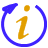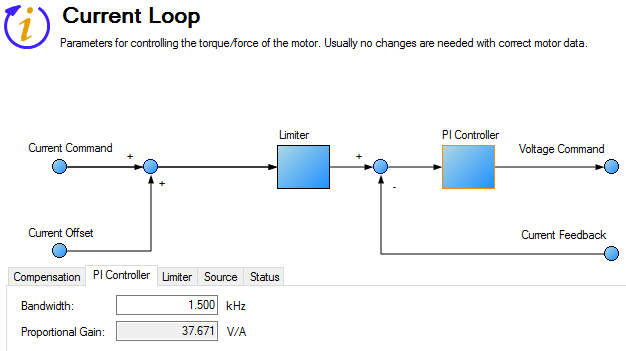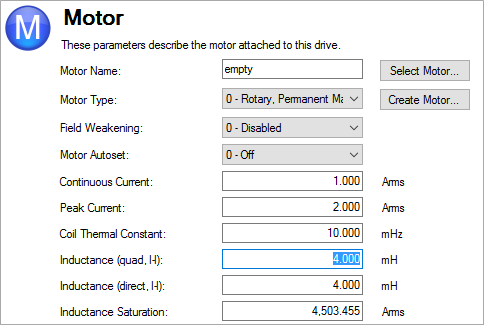#Current Loop

## Overview

The current loop controls the production of current on each axis. The parameters that govern the current loop are shown in the Current Loop view. The various types of tuning for the drive adjust these parameters automatically, so you normally do not need to adjust the current loop parameters in the current loop screen. The Current Loop view includes an active block diagram. If you click on a block in the diagram, the appropriate tab opens below.Element Property Description Parameter
Compensation Tab – items found here are a part of blocks #1 and #5 above
Offset Current Offset A constant current command added to compensate for gravity. AXIS#.IL.OFFSET
Friction Friction Current This is a friction compensation value. AXIS#.IL.FRICTION
Viscous FF Gain Current loop velocity feed-forward gain. AXIS#.IL.KVFF
Acceleration Feedforward Sets the gain for the acceleration feedforward (a scaled second derivative of the position command is added to the current command value) AXIS#.IL.KACCFF
PI Controller Tab – items found here are calculated in #3 above
Bandwidth Sets the current loop bandwidth. AXIS#.IL.BW
Proportional Gain Gets the proportional gain of the q-component of the PI regulator. This is a read-only value. AXIS#.IL.KP
Limiter Tab – items found here are calculated in #2 above
Positive Peak Sets the positive user (application-specific) current limit. AXIS#.IL.LIMITP
Negative Peak Sets the negative user (application-specific) current limit. AXIS#.IL.LIMITN
Source Tab
Commutation Source Tells the drive which feedback the Current Loop is using for commutation. AXIS#.IL.FBSOURCE
Analog Source Tells the drive which analog input the Current Loop is using for analog scaling. AXIS#.IL.AINSOURCE
Scale This item sets the analog current scale factor that scales the analog input. The value entered is the ratio of motor current per volt on analog input. AXIS#.IL.AINSCALE
Status Tab – items found here are calculated in #4, #6, and #7 above
Current Command Reads the value of the q-component current command. AXIS#.IL.CMD
Current Feedback Reads the actual value of the q-component current. AXIS#.IL.FB
Voltage Command Reads the output of the q-component PI regulator. AXIS#.IL.VCMD
U Winding Reads the sigma-delta measured current in the U-winding of the AXIS#.MOTOR. AXIS#.IL.IUFB
V Winding Reads the sigma-delta measured current in the V-winding of the AXIS#.MOTOR. AXIS#.IL.IVFB

## Current Loop Gain

The current loop bandwidth is defined by AXIS#.IL.BW. In most applications the default bandwidth value is acceptable.

The current loop gain AXIS#.IL.KP is based on the bandwidth and the motor inductance:

`AXIS#.IL.KP = 2 * pi * AXIS#.MOTOR.LQLL  * AXIS#.IL.BW.`

Where AXIS#.MOTOR.LQLL is the motor line-to-line inductance.The current loop gain is set automatically by the drive. The inductance used in the calculation is determined using one of the following methods:

• Option A. When AXIS#.MOTOR.AUTOSET = 1 and motor parameters are automatically identified on the AXIS#.IL.FBSOURCE feedback device, the proportional gain of the current loop (AXIS#.IL.KP ) is set based on AXIS#.MOTOR.LQLL read from motor memory. The gain is shown as a read-only parameter in the current loop screen.
• Option B.When the motor is selected using the motor database or using the custom motor tool, the specified Inductance (quad) value (AXIS#.MOTOR.LQLL ) is used to set the current loop proportional gain (AXIS#.IL.KP ).Related Parameters